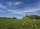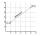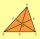# Right angled triangle 2

LMN is a right angled triangle with vertices at L(1,3), M(3,5) and N(6,n). Given angle LMN is 90° find n

Result

n =  2

#### Solution:Try calculation via our triangle calculator.

Leave us a comment of example and its solution (i.e. if it is still somewhat unclear...):

Showing 0 comments:Be the first to comment!#### To solve this example are needed these knowledge from mathematics:

For Basic calculations in analytic geometry is helpful line slope calculator. From coordinates of two points in the plane it calculate slope, normal and parametric line equation(s), slope, directional angle, direction vector, the length of segment, intersections the coordinate axes etc. Looking for help with calculating roots of a quadratic equation? Do you have a linear equation or system of equations and looking for its solution? Or do you have quadratic equation? Pythagorean theorem is the base for the right triangle calculator. See also our trigonometric triangle calculator.

## Next similar examples:

1. Triangle IRTIn isosceles right triangle ABC with right angle at vertex C is coordinates: A (-1, 2); C (-5, -2) Calculate the length of segment AB.
2. Vertices of RTShow that the points P1 (5,0), P2 (2,1) & P3 (4,7) are the vertices of a right triangle.
3. On lineOn line p: x = 4 + t, y = 3 + 2t, t is R, find point C, which has the same distance from points A [1,2] and B [-1,0].
4. Distance problem 2A=(x,2x) B=(2x,1) Distance AB=√2, find value of x
5. CathetiThe hypotenuse of a right triangle is 41 and the sum of legs is 49. Calculate the length of its legs.
6. DistanceCalculate distance between two points X[18; 19] and W[20; 3].
7. Isosceles triangleThe leg of the isosceles triangle is 5 dm, its height is 20 cm longer than the base. Calculate base length z.
8. Three pointsThree points A (-3;-5) B (9;-10) and C (2;k) . AB=AC What is value of k?
9. CenterIn the triangle ABC is point D[1,-2,6], which is the center of the |BC| and point G[8,1,-3], which is the center of gravity of the triangle. Find the coordinates of the vertex A[x,y,z].
10. Reference angleFind the reference angle of each angle:
11. Quadratic equationFind the roots of the quadratic equation: 3x2-4x + (-4) = 0.
12. Theorem proveWe want to prove the sentense: If the natural number n is divisible by six, then n is divisible by three. From what assumption we started?
13. DiscriminantDetermine the discriminant of the equation: ?
14. Solve 3Solve quadratic equation: (6n+1) (4n-1) = 3n2
15. EquationEquation ? has one root x1 = 8. Determine the coefficient b and the second root x2.
16. RootsDetermine the quadratic equation absolute coefficient q, that the equation has a real double root and the root x calculate: ?
17. LineIt is true that the lines that do not intersect are parallel?